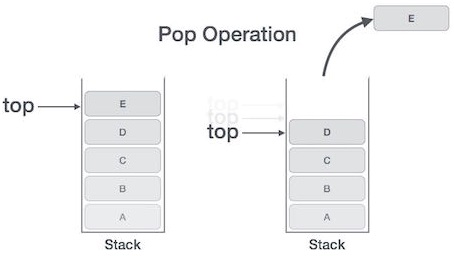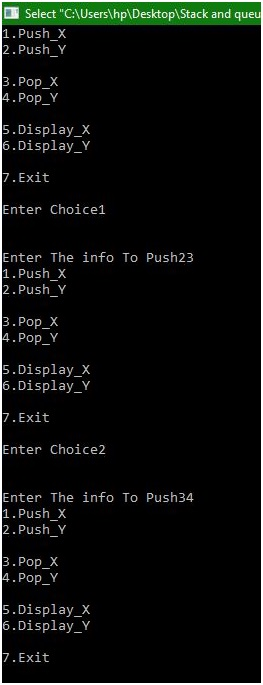# Implementation of Multi Stack in C

In this article, we are going to learn how to work more on than one stack by implementing multi stack in data structure?
Submitted by Manu Jemini, on December 17, 2017

We can manage multiple arrays to store different type of data and still manage them with a single index. A stack is a very important data structure because it can store data in a very practical way. Stack is a linear data structure. Stack array list fallow the Last In First Out principle. Element gets add up at the TOP and deleted from the TOP.In the Code below there are seven parts. Six functions are to implement three different operations like Insert an item, delete an item and display the Stack. The seventh function is the main function, in that a do while loop is implemented to keep the user engaged and provide him the all the given choices, according to the choice one of the six function get called.

The Push functions check whether the corresponding stack is full or not. If the stack is full it’s not do anything but if not it takes input from the user and push it to the top of the stack.

The Pop function checks whether the corresponding stack is empty, if not then it prints the topmost item and deletes it from the stack.

The Third function will simply print all the elements of the corresponding array if exist. If not, then it will say stack is Empty.

Note: The Stacks can hold only 5 items, for changing the capacity edit the third and fourth line.

### C program to implement multi stack

```#include<stdio.h>
#include<conio.h>
#define MAX_X 5
#define MAX_Y 5
int topx=-1;
int topy=10;

/*Begin of push_x*/
void push_x(int *stack)
{
int info;

if(topx>=(MAX_X-1))
{	printf("\n\nStack OverFlow");
return;
}
else
{	printf("\n\nEnter The info To Push");
scanf("%d",&info);
topx++;
stack[topx]=info;
}}
/*End of push_x*/
/*Begin of push_y*/
void push_y(int *stack)
{
int info;

if(topy<=(MAX_Y))
{
printf("\n\nStack OverFlow");
return;
}
else
{
printf("\n\nEnter The info To Push");
scanf("%d",&info);
topy--;
stack[topy]=info;
}
}
/*End of push_y*/
/*Begin of pop_x*/
void pop_x(int *stack)
{	if(topx==-1)
{
printf("Stack X is Underflow");
return;
}
else
{
printf("Item Poped from stack X is:%d\n",stack[topx]);

topx--;
}
}
/*End of pop_x*/
/*Begin of pop_y*/
void pop_y(int *stack)
{	if(topy==10)
{printf("Stack y is Underflow");
return;
}
else
{ printf("Item Poped from stack Y is:%d\n",stack[topy]);
topy++;
}}
/*End of pop_y*/
/*Begin of display_x*/
void display_x(int *stack)
{
int i;
if(topx==-1)
{
printf("Stack X is Empty");
return;
}
else
{ for(i=topx;i>=0;i--)
{printf("%d,",stack[i]);}
printf("\n");
}}
/*End of display_x*/
/*Begin of display_y*/
void display_y(int *stack)
{
int i;
if(topy==10)
{printf("Stack Y is Empty");
return;}
else
{for(i=topy;i<=9;i++)
{
printf("%d,",stack[i]);
}
printf("\n");
}	}
/*End of display_y*/
/*Begin of main*/
main()
{	int choice;
char ch;
int stack[MAX_X+MAX_Y];
do
{   printf("1.Push_X\n2.Push_Y\n");
printf("\n3.Pop_X\n4.Pop_Y\n");
printf("\n5.Display_X\n6.Display_Y\n");
printf("\n7.Exit");
printf("\n\nEnter Choice");
scanf("%d",&choice);
switch(choice)
{
case 1: push_x(stack);break;
case 2: push_y(stack);break;

case 3: pop_x(stack);break;
case 4: pop_y(stack);break;

case 5: display_x(stack);break;
case 6: display_y(stack);break;
case 7: break;
default: printf("Wrong Option...");
}
}while(choice!=7);
}
/*End of main*/
```

OutputTop MCQs